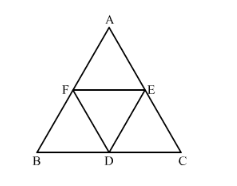# If D, E, F are the mid-points of sides BC, CA and AB respectively of ∆ABC, then the ratio of the areas of triangles DEF and ABC is

Question:

If D, E, F are the mid-points of sides BC, CA and AB respectively of ∆ABC, then the ratio of the areas of triangles DEF and ABC is

(a) 1 : 4
(b) 1 : 2
(c) 2 : 3
(d) 4 : 5

Solution:

GIVEN: In ΔABC, D, E and F are the midpoints of BC, CA, and AB respectively.

TO FIND: Ratio of the areas of ΔDEF and ΔABC

Since it is given that D and, E are the midpoints of BC, and AC respectively.

Therefore DE || AB, DE || FA……(1)

Again it is given that D and, F are the midpoints of BC, and, AB respectively.

Therefore, DF || CA, DF || AE……(2)

From (1) and (2) we get AFDE is a parallelogram.

Similarly we can prove that BDEF is a parallelogram.

$\angle \mathrm{FDE}=\angle \mathrm{A}$ (Opposite angles of $\|^{\mathrm{em}} \mathrm{AFDE}$ )

$\angle \mathrm{DEF}=\angle \mathrm{B}$ (Opposite angles of $\|^{\mathrm{mm}} \mathrm{BDEF}$ )

$\Rightarrow \triangle \mathrm{ABC} \sim \triangle \mathrm{DEF}(\mathrm{AA}$ similarity criterion)We know that the ratio of areas of two similar triangles is equal to the ratio of squares of their corresponding sides.

$\frac{\operatorname{ar}(\Delta \mathrm{DEF})}{\operatorname{ar}(\triangle \mathrm{ABC})}=\left(\frac{\mathrm{DE}}{\mathrm{AB}}\right)^{2}$

$\frac{\operatorname{ar}(\Delta \mathrm{DEF})}{\operatorname{ar}(\Delta \mathrm{ABC})}=\left(\frac{\frac{1}{2}(\mathrm{AB})}{\mathrm{AB}}\right)^{2}\left(\right.$ Since $\left.\mathrm{DE}=\frac{1}{2} \mathrm{AB}\right)$

$\frac{\operatorname{ar}(\Delta \mathrm{DEF})}{\operatorname{ar}(\Delta \mathrm{ABC})}=\left(\frac{1}{4}\right)$

Hence the correct option is $(a)$.